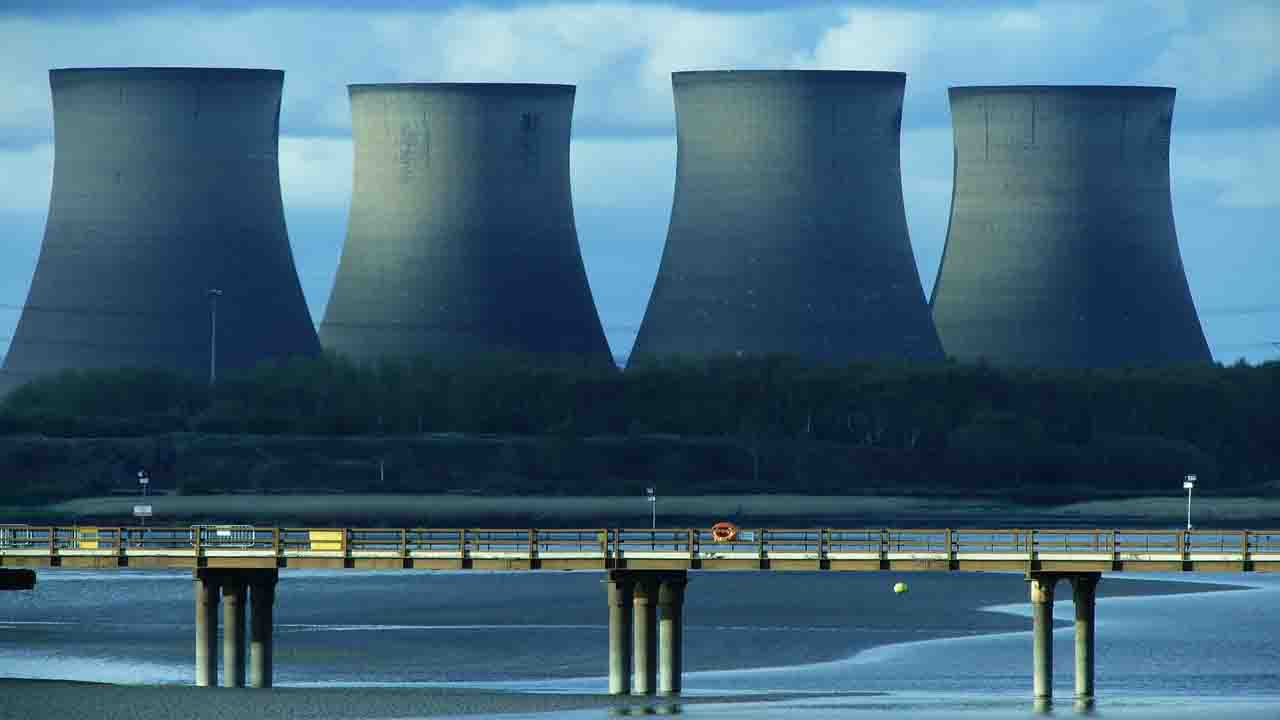# The Law of Conservation of Energy States that

0
3298# The Law of Conservation of Energy states that.

Energy can neither be created nor destroyed it can be converted from one form to another.

The law of conservation of energy is also called the first law of thermodynamics.

The first law of thermodynamics.

If a certain amount of heat is absorbed, an equivalent amount of work is done and if a certain amount of work is done, an equivalent amount of heat is produced.
1st law of thermodynamics examples.

When petrol or diesel burnt in a car engine, the chemical energy into this fuel converted into mechanical energy.

The solar panel converts radiation of the sun into electric energy.

Law of energy conservation.

This law states that the total energy of an isolated system remains constant, it is said to be conserved over time.

While the conservation of energy and conservation of mass is two different things.

But, the concept of special relativity showed that mass is related to energy and vice versa.

According to Einstein’s mass-energy relation E=mc².

Types of energy.Kinetic energy – The energy forms due to the motion.

Potential energy – The energy possessed by an object due to its position relative to other objects.

Chemical energy – The energy stored in chemical substances or bonds and which can be released in reactions.

Electrical energy – The energy stored in charged particles or separating charge in an electric field.

Thermal energy –  It is an example of kinetic energy, which can be transferred from one object or system to another in the form of heat. For example – friction.

## The second law of thermodynamics.

It is impossible to construct a heat engine operating in cycles, that converts whole heat into work.

This is a Planck-Kelvin statement of 2nd law of thermodynamics.

The second law of thermodynamics put some restrictions on the first law of thermodynamics.

This is because of the limitations of the first law of thermodynamics, such as the direction-flow of conversion and quantity of conversion.

2nd law of thermodynamics example.Before burned it a material, in a very ordered state.

But after burning more disordered state occurs, and you never combine, (heat and gases, etc.) these two states and cannot back to the form of an original piece of wood.

So you can say that an energy source is never destroyed but enters a disordered state and this state is not useful for us in terms of a source of energy.

“It means we can convert whole work in heat/energy but cannot convert whole heat into work done”.

Third law of thermodynamics.

Where the entropy measurement is zero, is a state of absolute zero temperature.

(Still today it is not possible by any procedure to achieve an absolute zero temp.)

Third law of thermodynamics example.
Entropy change of a crystal lattice heated by an incoming photon.
The source of this example is Wikipedia
That’s it all about “state the law of conservation of energy” in a simple way.
Some other related topics.